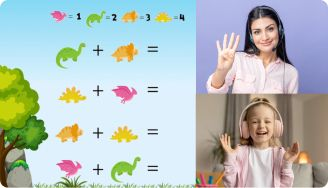# Number Line Worksheets

The number line worksheet is a math tool that has recently gained traction in education. This resource serves as a springboard for kids to learn other mathematical concepts. Worksheet problems comprise number line charting questions, word problems, questions requiring interpretation, and so on.

Leave your email and we will send you worksheets

worksheets sent successfully

## The benefits of the number line missing numbers worksheet

Students find number line worksheets helpful in visualizing mathematical concepts like addition and subtraction. The worksheet comprises numerous questions which students must answer. As kids solve these questions, they will learn the number line and its applications without difficulty.

1:1 Math Lessons

Want to raise a genius?
Start learning Math with BrighterlyThe worksheet’s questions have varying difficulty levels, which is helpful for knowledge advancement. With this arrangement, kids won’t be overwhelmed by the questions, and neither will they find them so easy that they lose interest in studying.

The format of the missing numbers on a number line worksheet ensures that students are not under any undue stress while they study. The worksheet contains real-life images and photos that will lock your kids’ attention to the pages. The benefits of using the worksheet are unsurprising since the worksheets are designed by professional tutors grounded in math and children’s education.

Learning with the number line worksheet also boosts critical skills in kids. It enhances their problem-solving abilities and reduces theшк fear of mathematics.

## Printable double number line worksheet PDF

Leave your email and we will send you worksheets

worksheets sent successfully### Number Line Worksheets PDF

View worksheet

Missing Numbers On A Number Line Worksheet### Number Line Worksheets PDF

View worksheet

Number Line Missing Numbers Worksheet### Number Line Worksheets PDF

View worksheet

Number Line Worksheet### Number Line Worksheets PDF

View worksheet

Rounding On A Number Line Worksheet

Book 1 to 1 Math Lesson• Specify your child’s math level
• Get practice worksheets for self-paced learning
• Your teacher sets up a personalized math learning plan for your child

Book 1 to 1 Math Lesson